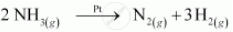Department of Pre-University Education, KarnatakaPUC Karnataka Science Class 12

# The decomposition of NH3 on platinum surface is zero order reaction. What are the rates of production - Chemistry

The decomposition of NH3 on platinum surface is zero order reaction. What are the rates of production of N2 and H2 if = 2.5 × 10−4 mol−1 L s−1?

#### Solution

The decomposition of NH3 on platinum surface is represented by the following equation.Therefore

Rate = -1/2 (d[NH_3])/dt = (d[N_2])/dt = 1/3(d[H_2])/dt

However, it is given that the reaction is of zero order.

Therefore,

-1/2 (d[NH_3])/dt = (d[N_2])/dt = 1/3 (d[H_2])/dt = k

= 2.5 x 10-4 mol L-1 s-1

Therefore, the rate of production of N2 is

(d[N_2])/dt = 2.5xx10^(-4) mol L^(-1) s^(-1)

And, the rate of production of H2 is

(d[H_2])/dt = 3 x 2.5 xx 10^(-4) mol L^(-1) s^(-1)

= 7.5 × 10−4 mol L−1 s−1

Concept: Integrated Rate Equations - Zero Order Reactions
Is there an error in this question or solution?
Chapter 4: Chemical Kinetics - Exercises [Page 117]

#### APPEARS IN

NCERT Class 12 Chemistry
Chapter 4 Chemical Kinetics
Exercises | Q 3 | Page 117
Share